# NCERT solutions for Class 9 Maths chapter 6 - Lines and Angles [Latest edition]

#### Chapters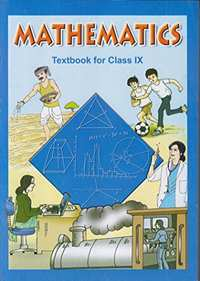## Solutions for Chapter 6: Lines and Angles

Below listed, you can find solutions for Chapter 6 of CBSE NCERT for Class 9 Maths.

Exercise 6.1Exercise 6.2Exercise 6.3
Exercise 6.1 [Pages 96 - 97]

### NCERT solutions for Class 9 Maths Chapter 6 Lines and Angles Exercise 6.1 [Pages 96 - 97]

Exercise 6.1 | Q 1 | Page 96

In the given figure, lines AB and CD intersect at O. If ∠AOC + ∠BOE = 70° and ∠BOD = 40°, find ∠BOE and reflex ∠COE.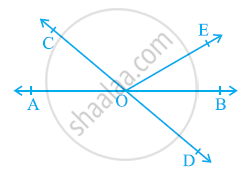Exercise 6.1 | Q 2 | Page 97

In the given figure, lines XY and MN intersect at O. If ∠POY = 90° and a:b = 2 : 3, find c.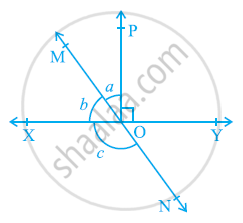Exercise 6.1 | Q 3 | Page 97

In the given figure, ∠PQR = ∠PRQ, then prove that ∠PQS = ∠PRT.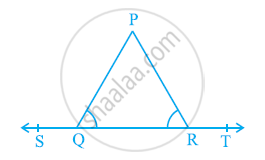Exercise 6.1 | Q 4 | Page 97

In the given figure, if x + y = w + z, then prove that AOB is a line.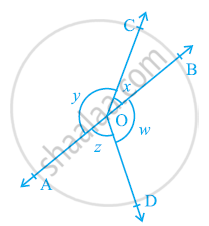Exercise 6.1 | Q 5 | Page 97

In the given figure, POQ is a line. Ray OR is perpendicular to line PQ. OS is another ray lying between rays OP and OR. Prove that ∠ROS = 1/2(∠QOS - ∠POS).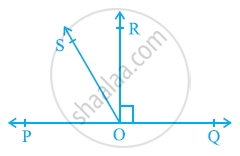Exercise 6.1 | Q 6 | Page 97

It is given that ∠XYZ = 64° and XY is produced to point P. Draw a figure from the given information. If ray YQ bisects ∠ZYP, find ∠XYQ and reflex ∠QYP.

Exercise 6.2 [Pages 103 - 105]

### NCERT solutions for Class 9 Maths Chapter 6 Lines and Angles Exercise 6.2 [Pages 103 - 105]

Exercise 6.2 | Q 1 | Page 103

In the given figure, find the values of x and y and then show that AB || CD.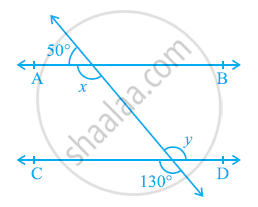Exercise 6.2 | Q 2 | Page 104

In the given figure, if AB || CD, CD || EF and y: z = 3: 7, find x.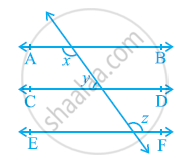Exercise 6.2 | Q 3 | Page 105

In the given figure, If AB || CD, EF ⊥ CD and ∠GED = 126º, find ∠AGE, ∠GEF and ∠FGE.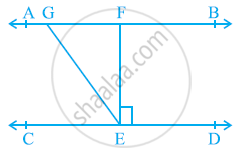Exercise 6.2 | Q 4 | Page 105

In the given figure, if PQ || ST, ∠PQR = 110º and ∠RST = 130º, find ∠QRS.

[Hint: Draw a line parallel to ST through point R.]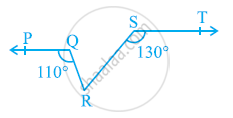Exercise 6.2 | Q 5 | Page 105

In the given figure, if AB || CD, ∠APQ = 50º and ∠PRD = 127º, find x and y.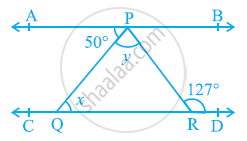Exercise 6.2 | Q 6 | Page 105

In the given figure, PQ and RS are two mirrors placed parallel to each other. An incident ray AB strikes the mirror PQ at B, the reflected ray moves along the path BC and strikes the mirror RS at C and again reflects back along CD. Prove that AB || CD.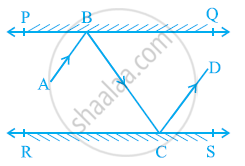Exercise 6.3 [Pages 107 - 108]

### NCERT solutions for Class 9 Maths Chapter 6 Lines and Angles Exercise 6.3 [Pages 107 - 108]

Exercise 6.3 | Q 1 | Page 107

In the given figure, sides QP and RQ of ΔPQR are produced to points S and T respectively. If ∠SPR = 135º and ∠PQT = 110º, find ∠PRQ.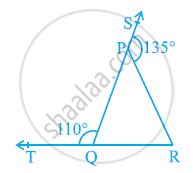Exercise 6.3 | Q 2 | Page 107

In the given figure, ∠X = 62º, ∠XYZ = 54º. If YO and ZO are the bisectors of ∠XYZ and ∠XZY respectively of ΔXYZ, find ∠OZY and ∠YOZ.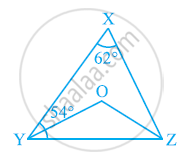Exercise 6.3 | Q 3 | Page 107

In the given figure, if AB || DE, ∠BAC = 35º and ∠CDE = 53º, find ∠DCE.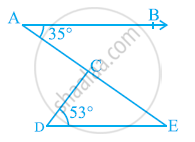Exercise 6.3 | Q 4 | Page 107

In the given figure, if lines PQ and RS intersect at point T, such that ∠PRT = 40º, ∠RPT = 95º and ∠TSQ = 75º, find ∠SQT.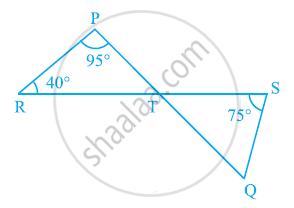Exercise 6.3 | Q 5 | Page 108

In the given figure, if PQ ⊥ PS, PQ || SR, ∠SQR = 28º and ∠QRT = 65º, then find the values of x and y.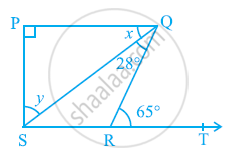Exercise 6.3 | Q 6 | Page 108

In the given figure, the side QR of ΔPQR is produced to a point S. If the bisectors of ∠PQR and ∠PRS meet at point T, then prove that ∠QTR = 1/2∠QPR.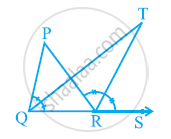## Solutions for Chapter 6: Lines and Angles

Exercise 6.1Exercise 6.2Exercise 6.3## NCERT solutions for Class 9 Maths chapter 6 - Lines and Angles

Shaalaa.com has the CBSE Mathematics Class 9 Maths CBSE solutions in a manner that help students grasp basic concepts better and faster. The detailed, step-by-step solutions will help you understand the concepts better and clarify any confusion. NCERT solutions for Mathematics Class 9 Maths CBSE 6 (Lines and Angles) include all questions with answers and detailed explanations. This will clear students' doubts about questions and improve their application skills while preparing for board exams.

Further, we at Shaalaa.com provide such solutions so students can prepare for written exams. NCERT textbook solutions can be a core help for self-study and provide excellent self-help guidance for students.

Concepts covered in Class 9 Maths chapter 6 Lines and Angles are Intersecting Lines and Non-intersecting Lines, Introduction to Lines and Angles, Basic Terms and Definitions, Parallel Lines, Pairs of Angles, Parallel Lines and a Transversal, Lines Parallel to the Same Line, Angle Sum Property of a Triangle.

Using NCERT Class 9 Maths solutions Lines and Angles exercise by students is an easy way to prepare for the exams, as they involve solutions arranged chapter-wise and also page-wise. The questions involved in NCERT Solutions are essential questions that can be asked in the final exam. Maximum CBSE Class 9 Maths students prefer NCERT Textbook Solutions to score more in exams.

Get the free view of Chapter 6, Lines and Angles Class 9 Maths additional questions for Mathematics Class 9 Maths CBSE, and you can use Shaalaa.com to keep it handy for your exam preparation.

Share